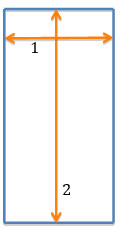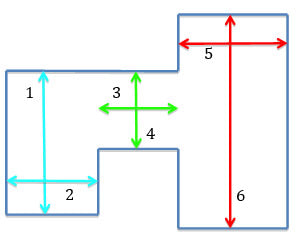# Measuring

In general measure the longest room length by the longest room width Figure 1, Length 1x Length 2 = Area 1In awkward shaped rooms, to be precise make a series of squares/rectangles within the room, calculate the area of each and add together, Figure 2.In Fig 2 six different measurements need to be taken, multiplied together ie:
Length 1x Length 2 = Area 1
Length 3x Length 4 = Area 2
Length 5x Length 6 = Area 3

Then add Area 1+ Area 2+ Area 3 = Total Area Required.